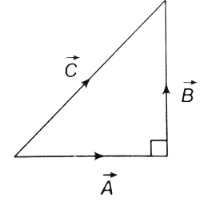# Addition and Subtraction of Vectors MCQs for NEET

Unlike scalars, vectors cannot be added algebraically because vectors possess both direction and magnitude. Following are a few points to note about vector addition:

• Addition of vectors implies finding the resultant of vectors acting on a body.
• Vectors, whose resultant is to be calculated behave independently of each other.
• The vectors can be added geometrically and not algebraically.

1. At what angle should the two force vectors 5N and 12 N be added to get a resultant vector of 13 N?

1. 00
2. 300
3. 900
4. 1800

2. If the sum of two unit vectors is 1, then what is the difference between them?

1. $$\frac{1}{\sqrt{2}}$$
2. $${\sqrt{2}}$$
3. $${\sqrt{3}}$$
4. $${\sqrt{4}}$$

Answer: (c) $${\sqrt{3}}$$

3. What is the net force on the particle if it is simultaneously acted upon by two forces 4 N and 3 N?

(a) 1 N

(b) 7 N

(c) 21 N

(d) Between 7 N and 1 N

Answer: (d) Between 7 N and 1 N

4. If the magnitude of the resultant force of two forces is lesser than the magnitude of the larger force, then

1. The two forces are perpendicular to each other
2. They two forces in opposite directions
3. The forces are different in magnitude and direction
4. The forces possess small magnitude

Answer: (b) The two forces are in opposite directions

5. Two forces $$\vec{F_1}$$ and $$\vec{F_2}$$ that are perpendicular to each other act on a point mass. The resultant force in the point mass is given by

1. $${F_1}+{F_2}$$
2. $${F_1}-{F_2}$$
3. $${F_1}^2+{F_2}^2$$
4. $$\sqrt{{F_1}^2+{F_2}^2}$$

Answer:(d) $$\sqrt{{F_1}^2+{F_2}^2}$$

6. If $$\left | \vec{A}-\vec{B}\right |=\left |\vec{A} \right |=\left |\vec{B} \right |$$, then what is the angle between $$\vec{A}$$ and $$\vec{B}$$?

1. 00
2. 300
3. 600
4. 900

7. Which of the following is true for the given diagram?1. $$\vec{A}+\vec{B}=\vec{C}$$
2. $$\vec{C}+\vec{A}=\vec{B}$$
3. $$\vec{B}+\vec{C}=\vec{A}$$
4. $$\vec{A}+\vec{B}+\vec{C}=0$$

Answer: (b) $$\vec{C}+\vec{A}=\vec{B}$$

8. If three vectors A, B and C have magnitudes 5, 12 and 13 and $$\vec{A}+\vec{B}=\vec{C}$$, then what is the angle between B and C?

1. $$cos^{-1}\frac{12}{13}$$
2. $$cos^{-1}\frac{13}{12}$$
3. $$cos^{-1}\frac{5}{7}$$
4. $$cos^{-1}\frac{7}{5}$$

Answer: (a) $$cos^{-1}\frac{12}{13}$$

9. Two forces F1 and F2 act on a body. One of the forces is double of the other force. The resultant of these forces is equal to the larger force. What is the angle between the forces?

1. $$cos^{-1}(\frac{1}{2})$$
2. $$cos^{-1}(-\frac{1}{2})$$
3. $$cos^{-1}(\frac{1}{4})$$
4. $$cos^{-1}(-\frac{1}{4})$$

Answer: (d) $$cos^{-1}(-\frac{1}{4})$$

10. A person travels 10 km North and 20 km East. What will be the displacement from the initial point?

1. 20 km
2. 22.36 km
3. 30 km
4. 32.36 km